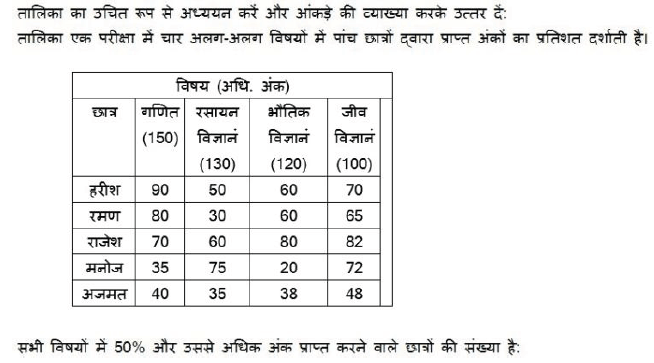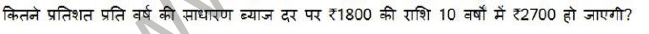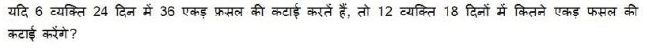# Math for SSC, RRB – Mock Test in Hindi| Online Exam Practice2Math Question Bank in Hindi for SSC, RRB – Mock Test

1 / 10

When a number is divided by 192, the remainder is 54. if the same number is divided by 16, what will be the remainder?

2 / 10

find the largest 6 digit number which is completely divided by 71 ?

3 / 10

The roman numeral form of 98 is

4 / 105 / 106 / 107 / 10

How many prime numbers are there between 1 and 100?

8 / 10

How many times the number 5 occur in the range of numbers from 1 to 100?

9 / 10

If n is divided by 6, the remainder is 4 . If 2n id divided by 6, then what will be the remainder?

10 / 10